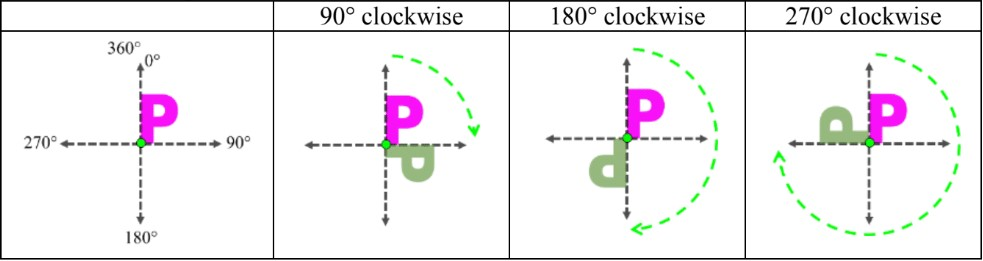# Reflections and rotations of shapes#### All in One Place

Everything you need for better grades in university, high school and elementary.#### Learn with Ease

Made in Canada with help for all provincial curriculums, so you can study in confidence.#### Instant and Unlimited Help

0/4
##### Intros
###### Lessons
1. Introduction to Reflections and Rotations of Shapes:
2. Drawing reflections (mirror images) on a grid
3. What is rotational symmetry?
4. Rotating shapes around a point (four main rotational angles)
5. Special example of reflecting and rotating a shape
0/18
##### Examples
###### Lessons
1. Drawing reflections on a grid - 1
Draw the mirror image after the shape is reflected across the mirror line (dotted line).
1.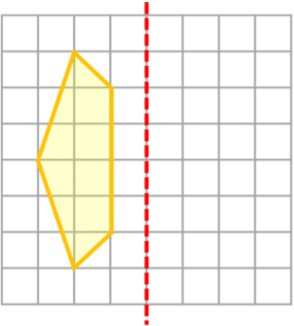2.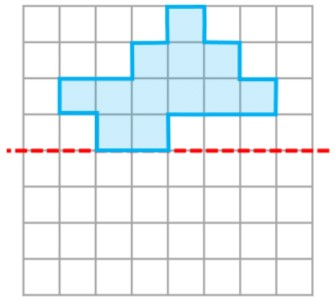3.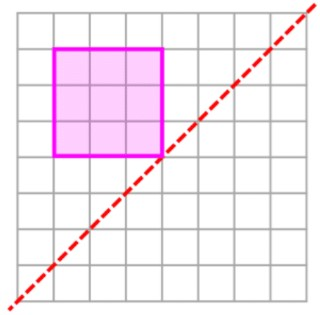2. Drawing reflections on a grid - 2
Draw the reflections of both the lines and the points across the mirror line (dotted line).
1.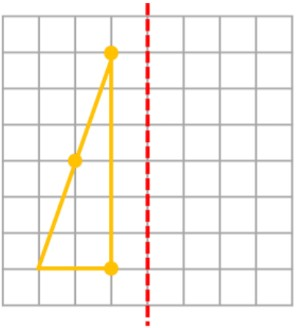2.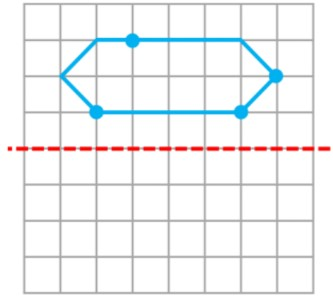3.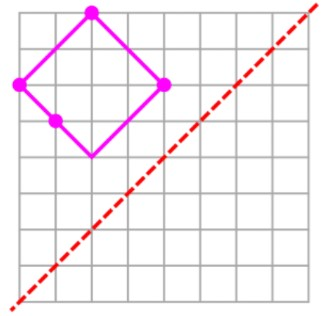3. Rotational symmetry
Does the picture have rotational symmetry? Yes or No.
1.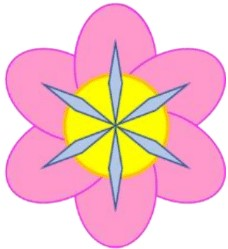2.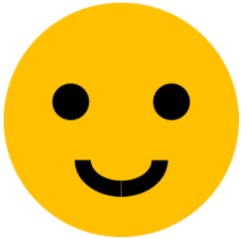3.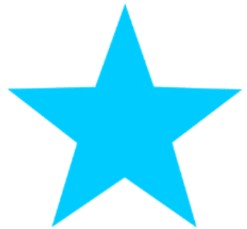4.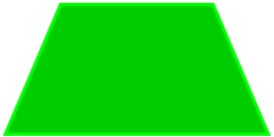4. Order of rotational symmetry
What is the order of rotational symmetry?
• How many times does the shape look the same in one rotation?
1.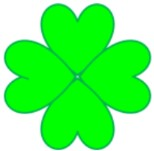2.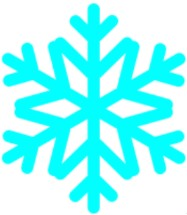3.5. Rotating shapes 90°, 180°, 270° or 360°
Draw what it will look like if you rotate the shape:
1.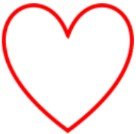1. 90° CW
2. 90° CCW
3. 180° CW
2.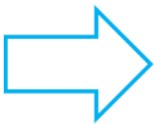1. 270° CW
2. 180° CCW
3. 360° CW
6. Recognizing reflections and rotations of shapes
Circle the pairs that are correct.
1. Which pairs are possible rotations of each other?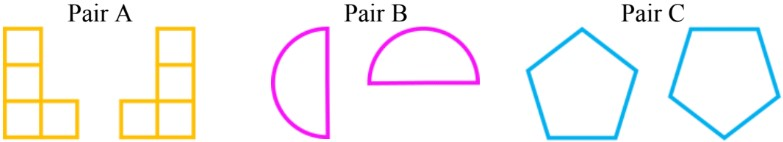2. Which pairs are possible reflections of each other?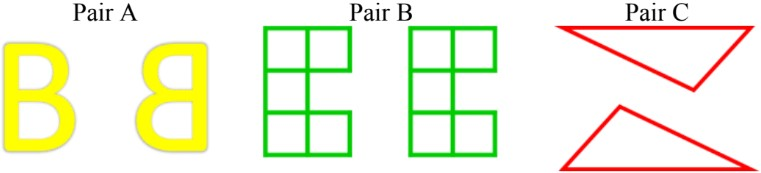3. Which pairs have the line of reflection drawn properly?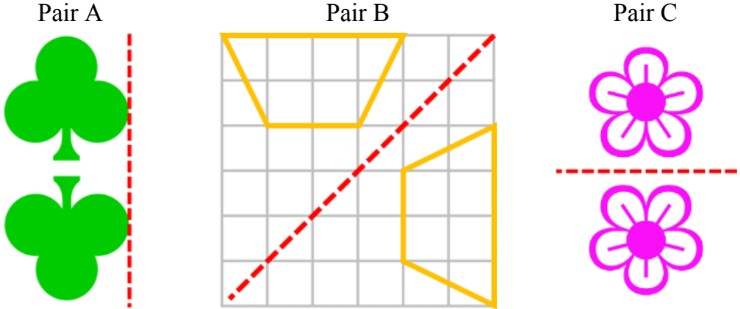###### Topic Notes

In this lesson, we will learn:

• How to draw reflections on a grid with vertical, horizontal, or diagonal mirror lines
• How to find order of rotational symmetry for shapes with multiple symmetry lines
• How to rotate shapes around a point 90°, 180°, 270°, and 360° clockwise or counter-clockwise

Notes:

• For line symmetry, a mirror test check if reflected halves match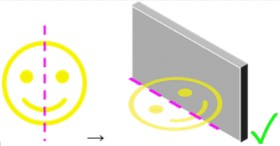• When drawing reflections of shapes, a grid and a line of symmetry are used
• For reflections, the line of symmetry will be called the “mirror line” instead
• There can be vertical, horizontal, and diagonal mirror lines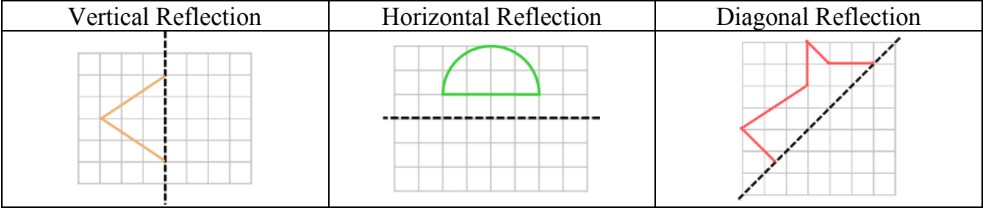• Choose some exact points of the original shape on the grid
• To draw the reflection of these points, they must be the same distance away from the mirror line as the original points (perpendicularly)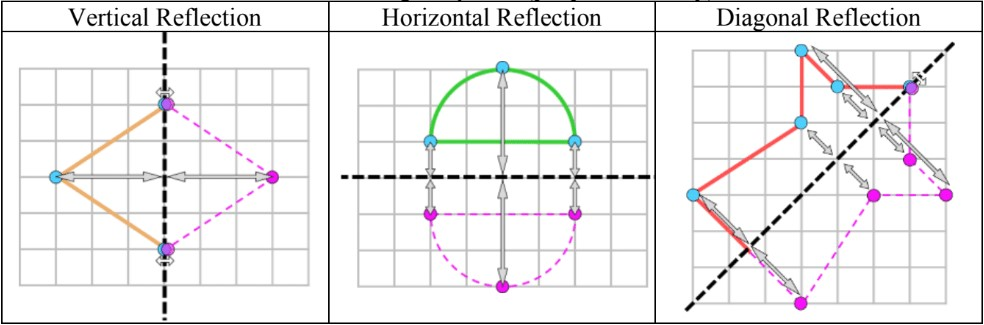• Another type of symmetry is called rotational symmetry
• If a shape has multiple lines of symmetry: when you spin it around in a full circle (360°), it will look exactly the same more than once

Ex. a triangle has 3 lines of symmetry; rotating a full circle, it matches the original 3 times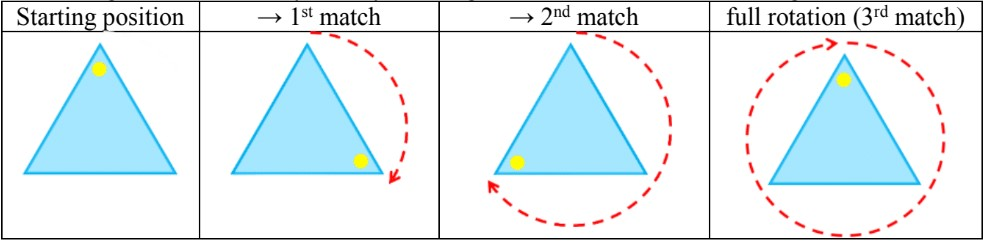• Therefore, the order of rotational symmetry for this regular triangle is = 3

• When rotating shapes, the shape will be spun around a point (usually the middle):
• either clockwise or counter-clockwise
• To one of 4 main rotation angles: 90°, 180°, 270°, and 360°

Ex. Rotate the shape of the letter “P” clockwise 90°, 180°, and 270° around the point